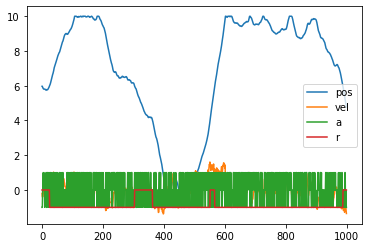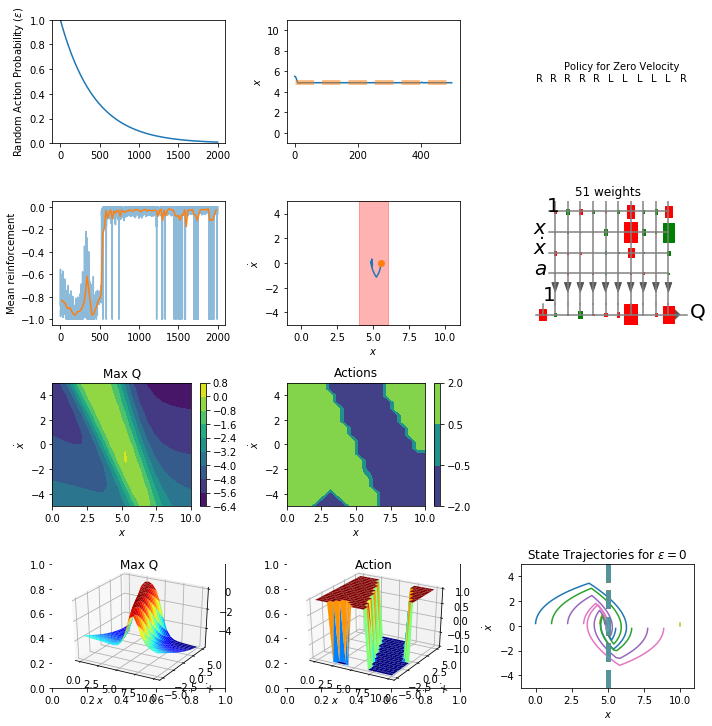$$\newcommand{\Rv}{\mathbf{R}} \newcommand{\rv}{\mathbf{r}} \newcommand{\Qv}{\mathbf{Q}} \newcommand{\Qnv}{\mathbf{Qn}} \newcommand{\Av}{\mathbf{A}} \newcommand{\Aiv}{\mathbf{Ai}} \newcommand{\av}{\mathbf{a}} \newcommand{\xv}{\mathbf{x}} \newcommand{\Xv}{\mathbf{X}} \newcommand{\yv}{\mathbf{y}} \newcommand{\Yv}{\mathbf{Y}} \newcommand{\zv}{\mathbf{z}} \newcommand{\av}{\mathbf{a}} \newcommand{\Wv}{\mathbf{W}} \newcommand{\wv}{\mathbf{w}} \newcommand{\betav}{\mathbf{\beta}} \newcommand{\gv}{\mathbf{g}} \newcommand{\Hv}{\mathbf{H}} \newcommand{\dv}{\mathbf{d}} \newcommand{\Vv}{\mathbf{V}} \newcommand{\vv}{\mathbf{v}} \newcommand{\Uv}{\mathbf{U}} \newcommand{\uv}{\mathbf{u}} \newcommand{\tv}{\mathbf{t}} \newcommand{\Tv}{\mathbf{T}} \newcommand{\TDv}{\mathbf{TD}} \newcommand{\Tiv}{\mathbf{Ti}} \newcommand{\Sv}{\mathbf{S}} \newcommand{\Gv}{\mathbf{G}} \newcommand{\zv}{\mathbf{z}} \newcommand{\Zv}{\mathbf{Z}} \newcommand{\Norm}{\mathcal{N}} \newcommand{\muv}{\boldsymbol{\mu}} \newcommand{\sigmav}{\boldsymbol{\sigma}} \newcommand{\phiv}{\boldsymbol{\phi}} \newcommand{\Phiv}{\boldsymbol{\Phi}} \newcommand{\Sigmav}{\boldsymbol{\Sigma}} \newcommand{\Lambdav}{\boldsymbol{\Lambda}} \newcommand{\half}{\frac{1}{2}} \newcommand{\argmax}{\underset{#1}{\operatorname{argmax}}} \newcommand{\argmin}{\underset{#1}{\operatorname{argmin}}} \newcommand{\dimensionbar}{\underset{#1}{\operatorname{|}}} \newcommand{\grad}{\mathbf{\nabla}} \newcommand{\ebx}{e^{\betav_{#1}^T \xv_n}} \newcommand{\eby}{e^{y_{n,#1}}} \newcommand{\Tiv}{\mathbf{Ti}} \newcommand{\Fv}{\mathbf{F}} \newcommand{\ones}{\mathbf{1}_{#1}}$$

# Reinforcement Learning to Control a Marble¶

Remember the one-dimensional maze example from last time.

We can make our one-dimensional maze problem into a realistic control problem by saying we have a marble on a one-dimensional track that has a mass and real-valued position and velocity values. So the state is actually two-dimensional, position, $x$, and velocity, $\dot{x}$. Actions are forces on the marble and let's just allow three forces, $-1$, $0$, and $1$.

First, download nnA4.zip. Unzip to get neuralnetwork_regresson.py. This is just like your A4 code with the addition of a draw function to show the structure of the network and its weights drawn as green and red rectangles.

In :
import numpy as np
import matplotlib.pyplot as plt
import neuralnetwork_regression as nn   # from A4


As before, we define the reinforcement, initial_state, and next_state functions to specify our dynamic marble problem.

$x_t$ is position in meters and $\dot{x_t}$ is velocity in meters / second

$$x_{t+k} = x_t + \int_{t'=t}^{t+k} \dot{x_{t'}} d{t'}$$$$x_{t+\Delta t} = x_t + \Delta t\; \dot{x_t}$$$$\dot{x}_{t+\Delta t} = \dot{x}_t + \Delta t\; \ddot{x_t}$$
In :
valid_actions = (-1, 0, 1)   # Possible actions

def reinforcement(s, sn):
goal = 5
return 0 if abs(sn[0 ]- goal) < 1 else -1
# return -abs(sn - goal)

def initial_state():
return np.array([10 * np.random.uniform(), 0.0])

def next_state(s, a):
''' is position, s is velocity. a is -1, 0 or 1'''
s = s.copy()
deltaT = 0.1                           # Euler integration time step
s += deltaT * s                  # Update position
force = a
mass = 0.5
s += deltaT * (force / mass - 0.2 * s)  # Update velocity. Includes friction

# Bound next position. If at limits, set velocity to 0.
if s < 0:
s = [0., 0.]    # these constants as ints were causing the errors we discussed in class. I DON'T KNOW WHY!!
elif s > 10:
s = [10., 0.]

return np.array(s)

In :
initial_state()

Out:
array([3.79705574, 0.        ])
In :
np.random.choice(valid_actions)

Out:
1
In :
s = initial_state()
pos = []
for i in range(1000):
a = np.random.choice(valid_actions)
s = next_state(s, a)
r = reinforcement(s, s)
pos.append(s.tolist() + [a, r])

plt.plot(np.array(pos))
plt.legend(('pos', 'vel', 'a', 'r'))

Out:
<matplotlib.legend.Legend at 0x7f0ad1aa31d0>In :
s = initial_state()
sn = next_state(s, -1)
reinforcement(s, sn)

Out:
-1

We also need our epsilon_greedy function.

In :
def epsilon_greedy(Qnet, state, valid_actions, epsilon):

if np.random.uniform() < epsilon:
# Random Move
action = np.random.choice(valid_actions)

else:
# Greedy Move
Qs = [Qnet.use(np.hstack((state, a)).reshape((1, -1))) for a in valid_actions]
ai = np.argmax(Qs)
action = valid_actions[ai]

Q = Qnet.use(np.hstack((state, action)).reshape((1, -1)))

return action, Q


The make_samples function is still general. We modify the one from the previous notes just a bit to allow our state variable to contain multiple values.

In :
def make_samples(Qnet, initial_state_f, next_state_f, reinforcement_f, valid_actions, n_samples, epsilon):

X = np.zeros((n_samples, Qnet.n_inputs))
R = np.zeros((n_samples, 1))
Qn = np.zeros((n_samples, 1))

s = initial_state_f()
s = next_state_f(s, 0)        # Update state, sn from s and a
a, _ = epsilon_greedy(Qnet, s, valid_actions, epsilon)

# Collect data from n_samples steps
for step in range(n_samples):

sn = next_state_f(s, a)        # Update state, sn from s and a
rn = reinforcement_f(s, sn)   # Calculate resulting reinforcement
an, qn = epsilon_greedy(Qnet, sn, valid_actions, epsilon) # Forward pass for time t+1
X[step, :] = np.hstack((s, a))
R[step, 0] = rn
Qn[step, 0] = qn
s, a = sn, an

return (X, R, Qn)


Like before, let's make a plot_status function to show us how we are doing.

In :
from mpl_toolkits.mplot3d import Axes3D
from matplotlib import cm

def plot_status(Qnet, trial, epsilon_trace, r_trace):

plt.subplot(4, 3, 1)
plt.plot(epsilon_trace[:trial + 1])
plt.ylabel('Random Action Probability ($\epsilon$)')
plt.ylim(0, 1)

plt.subplot(4, 3, 2)
plt.plot(X[:, 0])
plt.plot([0, X.shape], [5, 5], '--', alpha=0.5, lw=5)
plt.ylabel('$x$')
plt.ylim(-1, 11)
qs = Qnet.use(np.array([[s, 0, a] for a in valid_actions for s in range(11)]))

plt.subplot(4, 3, 3)
acts = ['L', '0', 'R']
actsiByState = np.argmax(qs.reshape((len(valid_actions), -1)), axis=0)
for i in range(11):
plt.text(i, 0, acts[actsiByState[i]])
plt.xlim(-1, 11)
plt.ylim(-1, 1)
plt.text(2, 0.2,'Policy for Zero Velocity')
plt.axis('off')

plt.subplot(4, 3, 4)
plt.plot(r_trace[:trial + 1], alpha=0.5)
binSize = 20
if trial+1 > binSize:
# Calculate mean of every bin of binSize reinforcement values
smoothed = np.mean(r_trace[:int(trial / binSize) * binSize].reshape((int(trial / binSize), binSize)), axis=1)
plt.plot(np.arange(1, 1 + int(trial / binSize)) * binSize, smoothed)
plt.ylabel('Mean reinforcement')

plt.subplot(4, 3, 5)
plt.plot(X[:, 0], X[: ,1])
plt.plot(X[0, 0], X[0, 1], 'o')
plt.xlabel('$x$')
plt.ylabel('$\dot{x}$')
plt.fill_between([4, 6], [-5, -5], [5, 5], color='red', alpha=0.3)  # CHECK OUT THIS FUNCTION!
plt.xlim(-1, 11)
plt.ylim(-5, 5)

plt.subplot(4, 3, 6)
Qnet.draw(['$x$', '$\dot{x}$', '$a$'], ['Q'])

plt.subplot(4, 3, 7)
n = 20
positions = np.linspace(0, 10, n)
velocities =  np.linspace(-5, 5, n)
xs, ys = np.meshgrid(positions, velocities)
xsflat = xs.flat
ysflat = ys.flat
qs = Qnet.use(np.array([[xsflat[i], ysflat[i], a] for a in valid_actions for i in range(len(xsflat))]))
qs = qs.reshape((len(valid_actions), -1)).T
qsmax = np.max(qs, axis=1).reshape(xs.shape)
cs = plt.contourf(xs, ys, qsmax)
plt.colorbar(cs)
plt.xlabel('$x$')
plt.ylabel('$\dot{x}$')
plt.title('Max Q')

plt.subplot(4, 3, 8)
acts = np.array(valid_actions)[np.argmax(qs, axis=1)].reshape(xs.shape)
cs = plt.contourf(xs, ys, acts, [-2, -0.5, 0.5, 2])
plt.colorbar(cs)
plt.xlabel('$x$')
plt.ylabel('$\dot{x}$')
plt.title('Actions')

s = plt.subplot(4, 3, 10)
rect = s.get_position()
ax = Axes3D(plt.gcf(), rect=rect)
ax.plot_surface(xs, ys, qsmax, cmap=cm.jet, linewidth=0)
ax.set_xlabel('$x$')
ax.set_ylabel('$\dot{x}$')
plt.title('Max Q')

s = plt.subplot(4, 3, 11)
rect = s.get_position()
ax = Axes3D(plt.gcf(), rect=rect)
ax.plot_surface(xs, ys, acts, cmap=cm.jet, linewidth=0)
ax.set_xlabel('$x$')
ax.set_ylabel('$\dot{x}$')
plt.title('Action')

plt.tight_layout()


And, let's write a function to plop the marble down at a number of positions and try to control it, plotting the results.

In :
def test_it(Qnet, n_trials, n_steps_per_trial):
xs = np.linspace(0, 10, n_trials)
plt.subplot(4, 3, 12)

# For a number (n_trials) of starting positions, run marble sim for n_steps_per_trial
for x in xs:

s = [x, 0]  # 0 velocity
x_trace = np.zeros((n_steps_per_trial, 2))
for step in range(n_steps_per_trial):
a, _ = epsilon_greedy(Qnet, s, valid_actions, 0.0) # epsilon = 0
s = next_state(s, a)
x_trace[step, :] = s

plt.plot(x_trace[:, 0], x_trace[:, 1])
plt.xlim(-1, 11)
plt.ylim(-5, 5)
plt.plot([5, 5], [-5, 5], '--', alpha=0.5, lw=5)
plt.ylabel('$\dot{x}$')
plt.xlabel('$x$')
plt.title('State Trajectories for $\epsilon=0$')

In :
def setup_standardization(Qnet, Xmeans, Xstds, Tmeans, Tstds):
Qnet.Xmeans = np.array(Xmeans)
Qnet.Xstds = np.array(Xstds)
Qnet.Tmeans = np.array(Tmeans)
Qnet.Tstds = np.array(Tstds)

In :
np.std([-1, 1]), np.std(np.arange(10))

Out:
(1.0, 2.8722813232690143)
In :
from IPython.display import display, clear_output
fig = plt.figure(figsize=(10, 10))

gamma = 0.8        # discount factor
n_trials = 2000         # number of repetitions of makeSamples-updateQ loop
n_steps_per_trial = 500 # number of steps between new random initial states
n_epochs = 10
learning_rate = 0.05
final_epsilon = 0.01 # value of epsilon at end of simulation. Decay rate is calculated
epsilon_decay =  np.exp(np.log(final_epsilon) / (n_trials)) # to produce this final value
print('epsilon_decay is', epsilon_decay)
epsilon_trace = np.zeros(n_trials)
r_trace = np.zeros(n_trials)

# Create the Qnet
nh =   # hidden layers structure
Qnet = nn.NeuralNetwork(3, nh, 1)
# Inputs are position (1 to 10) velocity (-3 to 3) and action (-1, 0, or 1)
setup_standardization(Qnet, [5, 0, 0], [2, 2, 0.8], [-2], )

epsilon = 1         # initial epsilon value

# Train for n_trials
for trial in range(n_trials):

# Collect n_steps_per_trial samples of X, R, Qn, and Q, and update epsilon
X, R, Qn = make_samples(Qnet, initial_state, next_state, reinforcement, valid_actions,
n_steps_per_trial, epsilon)

Qnet.train(X, R + gamma * Qn, n_epochs, learning_rate, method='sgd', verbose=False)

# Rest is for plotting
epsilon_trace[trial] = epsilon
epsilon *= epsilon_decay
r_trace[trial] = np.mean(R)
if True and (trial + 1 == n_trials or trial % (n_trials / 20) == 0):
fig.clf()
plot_status(Qnet, trial, epsilon_trace, r_trace)
test_it(Qnet, 10, 500)
clear_output(wait=True)
display(fig)

clear_output(wait=True)In [ ]:


In [ ]: